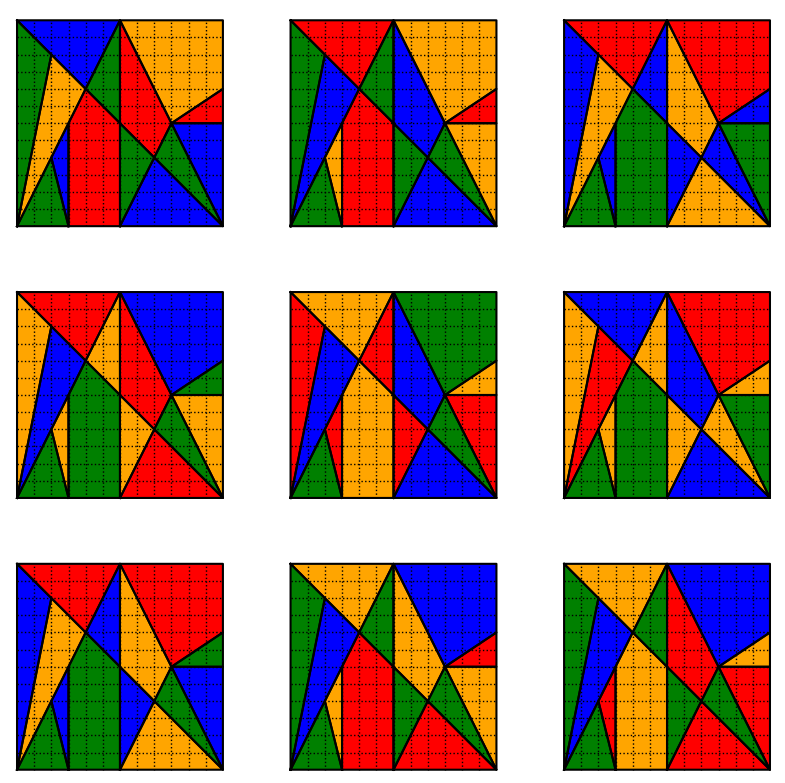# Ostomachion coloringThe following problems appeared in The Riddler. And it features an interesting combination of an ancient game with the four-color theorem.

The famous four-color theorem states, essentially, that you can color in the regions of any map using at most four colors in such a way that no neighboring regions share a color. A computer-based proof of the theorem was offered in 1976.

Some 2,200 years earlier, the legendary Greek mathematician Archimedes described something called an Ostomachion (shown below). It’s a group of pieces, similar to tangrams, that divides a 12-by-12 square into 14 regions. The object is to rearrange the pieces into interesting shapes, such as a Tyrannosaurus rex. It’s often called the oldest known mathematical puzzle.

Your challenge today: Color in the regions of the Ostomachion square with four colors such that each color shades an equal area. (That is, each color needs to shade 36 square units.) The coloring must also satisfy the constraint that no adjacent regions are the same color.

Extra credit: How many solutions to this challenge are there?Here are the details of how I solved the problem:
[Show Solution]

To jump straight to the solution (and pretty pictures!) see below.
[Show Solution]

## 3 thoughts on “Ostomachion coloring”

1.AVS says:

The small triangle in the lower-left corner is the same color (green) in all 44 solutions.
What do you make of that?

1.Laurent says:

Good observation! I counted two colorings as being the same solution if they had the same coloring pattern but with permuted colors. For example, if you had a shape with regions (R1,R2,R3,R4), and they were colored with (red,blue,green,blue), respectively, then this would be equivalent to the coloring (blue,green,red,green), since the defining characteristic of the first coloring is that R2 and R4 are the same color. Every 4-coloring belongs to a family of 24 equivalent colorings (since 4! = 24) which come from just permuting the colors.

In order to make the duplicate removal procedure more efficient so that I only count one of the 24 equivalent colorings for each solution, I constrained one of the triangles to always be green — precisely the triangle you pointed out.

2.AVS says:

Have you ever considered that the (O)Stomachion might be a 2-D rendering of a 3-D shape?